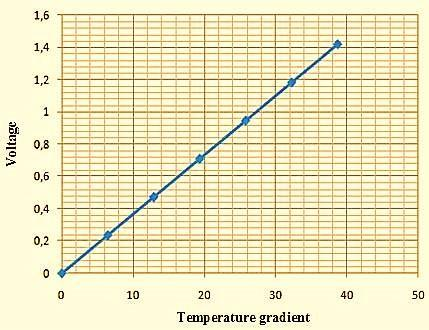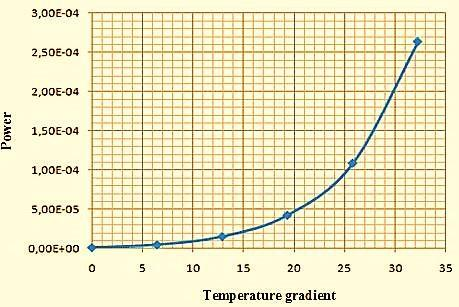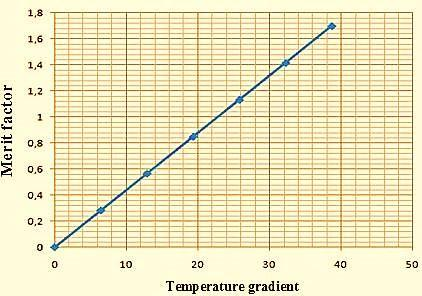# Analysis and Modeling of Thermoelectric Modules Based on Skutterudites Family

Analysis and Modeling of Thermoelectric Modules Based on Skutterudites Family

Ibtissem Sifi* Nassera Ghellai Younes Menni Ali J. Chamkha Houari Ameur

Unit of Research on Materials and Renewable Energies, Department of Physics, Faculty of Sciences, Abou Bekr Belkaid University, B.P. 119, 13000, Tlemcen, Algeria

Mechanical Engineering Department, Prince Sultan Endowment for Energy and Environment, Prince Mohammad Bin Fahd University, Al-Khobar 31952, Saudi Arabia

Department of Technology, University Center Salhi Ahmed of Naâma (Ctr Univ Naâma), B.P. 66, 45000, Naâma, Algeria

Corresponding Author Email:
ibtissemsifi2019@gmail.com
Page:
91-94
|
DOI:
https://doi.org/10.18280/ti-ijes.640114
10 October 2019
| |
Accepted:
15 January 2020
| | Citation

OPEN ACCESS

Abstract:

Thermoelectric modules are energy conversion devices which can either convert heat to electricity or operate in reverse as a heat pump. The physics of thermoelectricity can be modeled at several levels: quantum mechanical, statistical mechanical and at a macroscopic level using the transport equations directly. Among the new materials for the ambient temperature, one finds without being exhaustive the families of materials named ‘Skutterudites’, ‘Clathrates’ or ‘LAST’. According to composition, skutterudites can be of type ‘p’ or type ‘n’ but it is these which present the highest merit factors. Thus, ZT = 1.7 at T = 850 K was obtained in the Ba0,08La0,05Yb0,04Co4Sb12 structure case of the n type, while ZT = 1,16 at T 800 K in the Ba0,15Yb0,2In0,2Co4Sb12 structure case of the p type. The target of this analysis is to examine the characteristics of a new Ba0,08La0,05Yb0,04Co4Sb12 thermoelectric material, by MATLAB simulation.

Keywords:

analysis, modeling, simulation, thermoelectric modules, skutterudites family, energy conversion, lead antimony-silver-tellurium

1. Introduction

Many studies realized to examine the effect of thermoelectric generator (TEG) thermal physical characteristics on its efficiency. Terzioglu  analyzed the impact of the material on the performance of a TEG. The author used the approach of Taguchi to evaluate the TEG performance as a function of the material type. Cui et al.  reported a study of vibration with an analysis of strength failure of a TEG. Fan and Gao  numerically simulated the characteristics of a TEG with a segmented annular section for both the conditions, steady and transient. Li et al.  reported a theoretical model for a WCSPTEG. Lin et al.  addressed a novel configuration of TEG/TEC systems. Lv et al.  mathematically reported a model in order to enhance TEG efficiency. Merienne et al.  experimentally presented an analysis of the performance of generation of power of TEGs under the impact of heating rate. Ostrufka et al.  experimentally evaluated TEGs in nano-satellites. Shittu et al.  highlighted the characteristics of a SATEG using an analysis of finite elements. Wang et al.  used an analytical model to model the temperature and the performance of TEGs. Zhang et al.  addressed the impact of Thomson on the performance of a TEG using the approach of finite elements. Fernández-Yáñez et al.  analyzed the production of power for TEGs in the case of various contexts. Marvão et al.  optimized the TEG dimensions employing various algorithms. Abbasi and Tabar  measured and evaluated the TEG energy in vehicles. They also reported experimentally a prototype to validate their theoretical model. Abdo et al.  presented a novel type of PV/STEG hybrid system. This hybrid configuration has integrated by a MCHS. Angeline et al.  applied the ANN in order to simulate the efficiency of a HTEG by MATLAB. Asaadi et al.  numerically simulated the impact of various structural parameters on the thermodynamic characteristics of a two-stage ATEG. They also analyzed the exergoeconomic performance of this generator. Big-Alabo  experimentally evaluated the performance of a TEG using the Ge/SiGe structure. Bittner et al.  used materials of high performance to characterize the TEGs. Champier  reported a review study on the various applications of TEGs. Chen et al.  simulated the characteristics of TEGs in the cases of four various material structures. Choi et al.  experimentally analyzed the performance of porous media TEGs. Fan and Gao  reported a 3D numerical model of finite elements for ATE devices in order to improve their structural parameters. Karana and Sahoo  verified the impact of parameter of geometry on the characteristics of a novel ASTEG. Khanmohammadi and Saadat-Targhi  examined the impact of TEGMs station, inside a cycle of production of LNG, on the thermodynamic criteria and on the economic assessment. Other analyses can be found in previous studies [26-36]. Thermoelectric modules are energy conversion devices which can either convert heat to electricity or operate in reverse as a heat pump. The physics of thermoelectricity can be modeled at several levels: quantum mechanical, statistical mechanical and at a macroscopic level using the transport equations directly. Among the new materials for the ambient temperature, one finds without being exhaustive the families of materials named ‘Skutterudites’, ‘Clathrates’ or ‘LAST’. According to composition, skutterudites can be of type ‘p’ or type ‘n’ but it is these which present the highest merit factors. Thus, ZT = 1.7 at T = 850 K was obtained in the Ba0,08La0,05Yb0,04Co4Sb12 structure case of the n type, while ZT = 1,16 at T 800 K in the Ba0,15Yb0,2In0,2Co4Sb12 structure case of the p type. The target of this analysis is to examine the characteristics of a new Ba0,08La0,05Yb0,04Co4Sb12   thermoelectric material, by MATLAB simulation.

2. Governing Parameters

Seebeck Effect, S

$V=\left(S_{A}-S_{B}\right) \times\left(T_{1}-T_{2}\right)=S_{A B} \times \Delta T$           (1)

Peltier Effect, ᴨ

$d Q_{s}=\left(\Pi_{A}-\Pi_{B}\right) \vec{J} \cdot \overrightarrow{u_{A B}}$           (2)

Kelvin relations

$\Pi=S T$             (3)

$\frac{\partial S_{\mathrm{AB}}}{\partial \mathrm{T}}=\frac{\beta_{\mathrm{A}}-\beta_{\mathrm{B}}}{\mathrm{T}}$             (4)

Figure of merit, ZT

$Z T=\frac{\sigma T S^{2}}{\lambda}$            (5)

3. Results and Discussion

Figure 1 addresses the variation in the voltage (V), as a function of gradient of temperature. As expected, the average value of negative charge energy of electrons is very large at the level of the hot space compared to that on the cold space, due to the metal undergoes a thermal gradient, which leads to a gradient of energy, allowing the diffusion of electrons on the cold surface.

## 1.jpgFigure 1. V values as a function of gradient of temperature

Figure 2 reports the evolution of variation of current (I) as a function of gradient of temperature. If a current is passed through a circuit with two different conductors joining them at the same temperature, the thermal energy will be absorbed at the first junction, while it will be restored to the second. Let us now consider a and b as respectively n-type and p-type semiconductors.This diffusion leads to the polarization of the material (accumulation of negative majority carriers on the cold side and positive majority positive carriers on the warm side) which induces an electric field whose effect is to help the cold electrons to diffuse towards the hot side. Is what is causing the potential difference that appears on the thermoelectric generator.

## 2.jpgFigure 2. I values as a function of gradient of temperature

The junction who absorbs heat is then that in which the I flows from the first material in the type (n) to the second material in the type (p) is junction at T. Conversely, one that restores it is the junction in which the current flows from the material of the type p to the material of the type n, i.e., the junction with the temperature T. Thus, we have T > T1.

Figure 3 presents the distribution of the power (W) for different gradients of temperature (T). A TEG is a small module formed of two ceramic plates. It has appeared to us that the thermal flux inside such a TE module is the factor responsible for the production of electricity. That is, there must be heat transfer between the two ceramic plates.

## 3.jpgFigure 3. W values as a function of gradient of temperature

For case where one of them is of temperature higher than the second one there is thermal transfer, that is to say that the hottest body will supply energy to the coldest body, until reaching the thermal equilibrium.

Figure 4 shows the effect of gradient of temperature on the ZT value. The value of ZT defines whether the material has good thermoelectric properties or not.

## 4.jpgFigure 4. ZT values as a function of gradient of temperature

As is known, the best TE material has a high ZT value, better S value, improved current conductivity with week λ value. Best TEMs have ZT slightly greater than 1.7.

4. Conclusion

The target of this analysis is to examine the characteristics of a new Ba0,08La0,05Yb0,04Co4Sb12 thermoelectric material (TEM), by MATLAB simulation. The findings of this research may serve as

As expected, the average value of negative charge energy of electrons is very large at the level of the hot space compared to that on the cold space, due to the metal undergoes a thermal gradient, which leads to a gradient of energy, allowing the diffusion of electrons on the cold surface.

This diffusion leads to the polarization of the material (accumulation of negative majority carriers on the cold side and positive majority positive carriers on the warm side) which induces an electric field whose effect is to help the cold electrons to diffuse towards the hot side. Is what is causing the potential difference that appears on the thermoelectric generator.

If a current is passed through a circuit with two different conductors joining them at the same temperature, the thermal energy will be absorbed at the first junction, while it will be restored to the second. Let us now consider a and b as respectively n-type and p-type semiconductors.

The junction who absorbs heat is then that in which the I flows from the first material in the type (n) to the second material in the type (p) is junction at T. Conversely, one that restores it is the junction in which the current flows from the material of the type p to the material of the type n, i.e., the junction with the temperature T. Thus, we have T > T1.

A TEG is a small module formed of two ceramic plates. It has appeared to us that the thermal flux inside such a TE module is the factor responsible for the production of electricity. That is, there must be heat transfer between the two ceramic plates.

For case where one of them is of temperature higher than the second one there is thermal transfer, that is to say that the hottest body will supply energy to the coldest body, until reaching the thermal equilibrium.

As is known, the best TE material has a high ZT value, better S value, improved current conductivity with week λ value. Best TEMs have ZT slightly greater than 1.7.

References

 Terzioglu, H. (2020). Analysis of effect factors on thermoelectric generator using Taguchi method. Measurement, 149: 106992. https://doi.org/10.1016/j.measurement.2019.106992

 Cui, Y.J., Wang, B.L., Wang, K.F. (2019). Thermally induced vibration and strength failure analysis of thermoelectric generators. Applied Thermal Engineering, 160: 113991. https://doi.org/10.1016/j.applthermaleng.2019.113991

 Fan, S., Gao, Y. (2019). Numerical analysis on the segmented annular thermoelectric generator for waste heat recovery. Energy, 183: 35-47. https://doi.org/10.1016/j.energy.2019.06.103

 Li, G., Zheng, Y., Hu, J., Guo, W. (2019). Experiments and a simplified theoretical model for a water-cooled, stove-powered thermoelectric generator. Energy, 185: 437-448. https://doi.org/10.1016/j.energy.2019.07.023

 Lin, L., Zhang, Y.F., Liu, H.B., Meng, J.H., Chen, W.H., Wang, X.D. (2019). A new configuration design of thermoelectric cooler driven by thermoelectric generator. Applied Thermal Engineering, 160: 114087. https://doi.org/10.1016/j.applthermaleng.2019.114087

 Lv, S., He, W., Hu, Z., Liu, M., Qin, M., Shen, S., Gong, W. (2019). High-performance terrestrial solar thermoelectric generators without optical concentration for residential and commercial rooftops. Energy Conversion and Management, 196: 69-76. https://doi.org/10.1016/j.enconman.2019.05.089

 Merienne, R., Lynn, J., McSweeney, E., O'Shaughnessy, S.M. (2019). Thermal cycling of thermoelectric generators: The effect of heating rate. Applied Energy, 237: 671-681. https://doi.org/10.1016/j.apenergy.2019.01.041

 Ostrufka, A.L.A., Filho, E.M., Borba, A.C., Spengler, A.W., Possamai, T.S., Paiva, K.V. (2019). Experimental evaluation of thermoelectric generators for nano-satellites application. Acta Astronautica, 162: 32-40. https://doi.org/10.1016/j.actaastro.2019.05.053

 Shittu, S., Li, G., Zhao, X., Ma, X., Akhlaghi, Y.G., Ayodele, E. (2019). High performance and thermal stress analysis of a segmented annular thermoelectric generator. Energy Conversion and Management, 184: 180-193. https://doi.org/10.1016/j.enconman.2019.01.064

 Wang, P., Wang, B.L., Li, J.E. (2019). Temperature and performance modeling of thermoelectric generators. International Journal of Heat and Mass Transfer, 143: 118509. https://doi.org/10.1016/j.ijheatmasstransfer.2019.118509

 Zhang, M., Tian, Y., Xie, H., Wu, Z., Wang, Y. (2019). Influence of Thomson effect on the thermoelectric generator. International Journal of Heat and Mass Transfer, 137: 1183-1190. https://doi.org/10.1016/j.ijheatmasstransfer.2019.03.155

 Fernández-Yáñez, P., Armas, O., Kiwan, R., Stefanopoulou, A.G., Boehman, A.L. (2018). A thermoelectric generator in exhaust systems of spark-ignition and compression-ignition engines. A comparison with an electric turbo-generator. Applied Energy, 229: 80-87. https://doi.org/10.1016/j.apenergy.2018.07.107

 Marvão, A., Coelho, P.J., Rodrigues, H.C. (2019). Optimization of a thermoelectric generator for heavy-duty vehicles. Energy Conversion and Management, 179: 178-191. https://doi.org/10.1016/j.enconman.2018.10.045

 Abbasi, V., Tabar, V.S. (2020). Measurement and evaluation of produced energy by thermoelectric generator in vehicle. Measurement, 149: 107035.  https://doi.org/10.1016/j.measurement.2019.107035

 Abdo, A., Ookawara, S., Ahmed, M. (2019). Performance evaluation of a new design of concentrator photovoltaic and solar thermoelectric generator hybrid system. Energy Conversion and Management, 195: 1382-1401. https://doi.org/10.1016/j.enconman.2019.04.093

 Angeline, A.A., Asirvatham, L.G., Hemanth, D.J., Jayakumar, J., Wongwises, S. (2019). Performance prediction of hybrid thermoelectric generator with high accuracy using artificial neural networks. Sustainable Energy Technologies and Assessments, 33: 53-60. https://doi.org/10.1016/j.seta.2019.02.008

 Asaadi, S., Khalilarya, S., Jafarmadar, S. (2019). A thermodynamic and exergoeconomic numerical study of two-stage annular thermoelectric generator. Applied Thermal Engineering, 156: 371-381. https://doi.org/10.1016/j.applthermaleng.2019.04.058

 Big-Alabo, A. (2019). Performance evaluation of Ge/SiGe-based thermoelectric generator. Physica E: Low-dimensional Systems and Nanostructures, 108: 202-205. https://doi.org/10.1016/j.physe.2018.12.024

 Bittner, M., Kanas, N., Hinterding, R., Steinbach, F., Räthel, J., Schrade, M., Wiik, K., Einarsrud, M.A., Feldhoff, A. (2019). A comprehensive study on improved power materials for high-temperature thermoelectric generators. Journal of Power Sources, 410-411: 143-151. https://doi.org/10.1016/j.jpowsour.2018.10.0766

 Champier, D. (2017). Thermoelectric generators: a review of applications. Energy Conversion and Management, 140: 167-181. http://dx.doi.org/10.1016/j.enconman.2017.02.070

 Chen, W.H., Lin, Y.X., Wang, X.D., Lin, Y.L. (2019). A comprehensive analysis of the performance of thermoelectric generators with constant and variable properties. Applied Energy, 241: 11-24. https://doi.org/10.1016/j.apenergy.2019.02.083

 Choi, Y., Negash, A., Kim, T.Y. (2019). Waste heat recovery of diesel engine using porous medium-assisted. Energy Conversion and Management, 197: 111902. https://doi.org/10.1016/j.enconman.2019.111902

 Fan, S., Gao, Y. (2018). Numerical simulation on thermoelectric and mechanical performance of annular thermoelectric generator. Energy, 150: 38-48. https://doi.org/10.1016/j.energy.2018.02.124

 Karana, D.R., Sahoo, R.R. (2019). Influence of geometric parameter on the performance of a new asymmetrical and segmented thermoelectric generator. Energy, 179: 90-99. https://doi.org/10.1016/j.energy.2019.04.199

 Khanmohammadi, S., Saadat-Targhi, M. (2019). Thermodynamic and economic assessment of an integrated thermoelectric generator and the liquefied natural gas production process. Energy Conversion and Management, 185: 603-610. https://doi.org/10.1016/j.enconman.2019.02.034

 Makki, A., Omer, S., Su, Y., Sabir, H. (2016). Numerical investigation of heat pipe-based photovoltaic-thermoelectric generator (HP-PV/TEG) hybrid system. Energy Conversion and Management, 112: 274-287. http://dx.doi.org/10.1016/j.enconman.2015.12.069

 Elefsiniotis, A., Kokorakis, N., Becker, T., Schmid, U. (2014). A thermoelectric-based energy harvesting module with extended operational temperature range for powering autonomous wireless sensor nodes in aircraft. Sens Actuators, A, 206: 159-164. http://dx.doi.org/10.1016/j.sna.2013.11.036

 Lertsatitthanakorn, C., Khasee, N., Atthajariyakul, S., Soponronnarit, S., Therdyothin, A., Suzuki, R.O. (2008). Performance analysis of a double-pass thermoelectric solar air collector. Sol Energy Mater Sol Cells, 92: 1105-1109. http://dx.doi.org/10.1016/j.solmat.2008.03.018

 Lan, S., Smith, A., Stobart, R., Chen, R. (2019). Feasibility study on a vehicular thermoelectric generator for both waste heat recovery and engine oil warm-up. Applied Energy, 242(2019): 273-284. https://doi.org/10.1016/j.apenergy.2019.03.056

 Xie, Y., Wu, S., Yang, C. (2016). Generation of electricity from deep-sea hydrothermal vents with a thermoelectric converter. Appl Energy, 164: 620-627. http://dx.doi.org/10.1016/j.apenergy.2015.12.036

 Kraemer, D., McEnaney, K., Chiesa, M., Chen, G. (2012). Modeling and optimization of solar thermoelectric generators for terrestrial applications. Solar Energy, 86: 1338-1350. http://dx.doi.org/10.1016/j.solener.2012.01.025

 Leonov, V., Vullers, R.J.M., Hoof, C.V. (2012). Thermoelectric generator hidden in a shirt with a fabric radiator. AIP Conf Proc., 1449: 556-559. http://dx.doi.org/10.1063/1.4731617

 Baranowski, L.L., Snyder, G.J., Toberer, E.S. (2012). Concentrated solar thermoelectric generators. Energy Environ Sci., 5: 9055-9067. http://dx.doi.org/10.1039/C2EE22248E

 Chen, W.H., Wang, C.C., Hung, C.I., Yang, C.C., Juang, R.C. (2014). Modeling and simulation for the design of thermal-concentrated solar thermoelectric generator. Energy, 64: 287-297. http://dx.doi.org/10.1016/j.energy.2013.10.073

 He, W., Su, Y., Riffat, S.B., Hou, J., Ji, J. (2011). Parametrical analysis of the design and performance of a solar heat pipe thermoelectric generator unit. Appl Energy, 88: 5083-5089. http://dx.doi.org/10.1016/j.apenergy.2011.07.017

 Kossyvakis, D.N., Vossou, C.G., Provatidis, C.G., Hristoforou, E.V. (2015). Computational analysis and performance optimization of a solar thermoelectric generator. Renewable Energy, 81: 150-161. http://dx.doi.org/10.1016/j.renene.2015.03.026